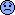programming forums Java Mobile Certification Databases Caching Books Engineering Micro Controllers OS Languages Paradigms IDEs Build Tools Frameworks Application Servers Open Source This Site Careers Other Pie Elite all forums
this forum made possible by our volunteer staff, including ...
Marshals:
• Campbell Ritchie
• Paul Clapham
• Ron McLeod
• Jeanne Boyarsky
• Tim Cooke
Sheriffs:
• Liutauras Vilda
• paul wheaton
• Henry Wong
Saloon Keepers:
• Tim Moores
• Tim Holloway
• Stephan van Hulst
• Carey Brown
• Frits Walraven
Bartenders:
• Piet Souris
• Himai Minh

# casting

Ranch Hand
Posts: 130
••Number of slices to send:
Optional 'thank-you' note:
••Please take a look at the following code.
CODE:
class Casting
{
public static void main(String args[])
{
int i=0;
long l=0;
short s=0;
float f = Float.NaN;
float f1 = Float.POSITIVE_INFINITY;
float f2 = Float.NEGATIVE_INFINITY;
i = (int)f1;
l= (long)f1;
s= (short)f1;
System.out.println("the value in i is:" + i ); //It's printing the largest representable value of type int.

System.out.println("the value in l is:" + l); //It's printing the largest representable value of type long.
System.out.println("the value in s is:" + s); //It's printing -1
}
}
Why s value is not the largest representable value of type short.
Thanks.[This message has been edited by sree (edited March 17, 2000).]

Ranch Hand
Posts: 1467
••Number of slices to send:
Optional 'thank-you' note:
••When you convert a float to short/byte/char , the float is first converted to an int and then it is again converted to the corresponding types.
So here when you convert Float.POSITIVE_INFINITY to short it is first converted maximum representable int value which is Integer.MAX_VALUE has the value of 0x7fff ffff
When you convert an int to short var, the last 2 bytes are truncated and assigned to the short var. So here we get 0xffff . Since short is signed primitive 0xffff gives value -1
regds
maha anna

[This message has been edited by maha anna (edited March 17, 2000).]

sreelakshmi sarma
Ranch Hand
Posts: 130
••Number of slices to send:
Optional 'thank-you' note:
••Thanks Anna. But there is still something that's bothering me. Let's say we change the above code like this.
code:
class Casting
{
public static void main(String args[])
{
short s=0;
double d=23456789.9900;
s= (short)d;
System.out.println("the value of s is" + s);
}
}
If there are couple of choices and i have to choose one how will i know which one is the right one.
Thanks.

maha anna
Ranch Hand
Posts: 1467
••Number of slices to send:
Optional 'thank-you' note:
••The rules are these from JLS. Read this carefully. You can go from there.
regds
maha anna
A narrowing conversion of a floating-point number to an integral type T takes two steps:
In the first step, the floating-point number is converted either to a long, if T is long, or to an int, if T is byte, short, char, or int, as follows:
If the floating-point number is NaN , the result of the first step of the conversion is an int or long 0.
Otherwise, if the floating-point number is not an infinity, the floating-point value is rounded to an integer value V, rounding toward zero using IEEE 754 round-toward-zero mode . Then there are two cases:
If T is long, and this integer value can be represented as a long, then the result of the first step is the long value V.
Otherwise, if this integer value can be represented as an int, then the result of the first step is the int value V.
Otherwise, one of the following two cases must be true:
The value must be too small (a negative value of large magnitude or negative infinity), and the result of the first step is the smallest representable value of type int or long.
The value must be too large (a positive value of large magnitude or positive infinity), and the result of the first step is the largest representable value of type int or long.
In the second step:
If T is int or long, the result of the conversion is the result of the first step.
If T is byte, char, or short, the result of the conversion is the result of a narrowing conversion to type T of the result of the first step.

[This message has been edited by maha anna (edited March 17, 2000).]She's out of the country right now, toppling an unauthorized dictatorship. Please leave a message with this tiny ad: Free, earth friendly heat - from the CodeRanch trailboss https://www.kickstarter.com/projects/paulwheaton/free-heat